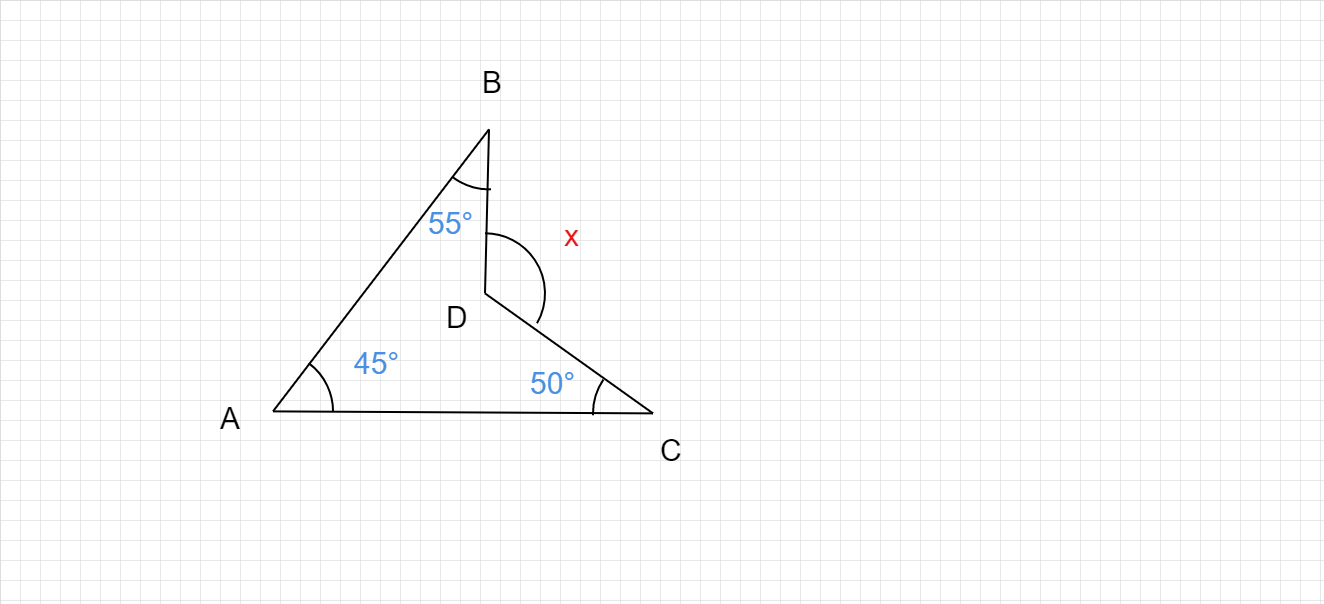"
">

# Find the value of x from the following figure :"

Given :

To do :

We have to find the value of x.

Solution :

$$Sum of the angles in a quadrilateral is 360°$$.

Therefore,

$45°+50°+55°+ ∠ADC = 360°$          [Here, $∠ADC$ is the angle opposite to $∠x$]

$∠ADC = 360° - 150°$

$∠ADC = 210°$

We know that,

Sum of the angles about a point is 360° .

Therefore,

$∠ADC+∠x = 360°$

$210° + ∠x = 360°$

$∠x = 360° -210°$

$∠x = 150°$.

The measure of angle x is 150°.

Updated on: 10-Oct-2022

34 Views